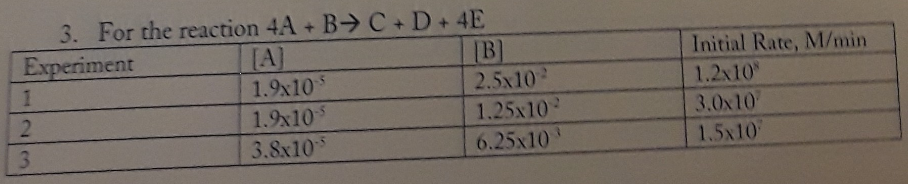# Rate Law: For the following problem, determine the rate law (find order) for the stated reaction, write the rate law, and determine the value and units of the rate constant. For the reaction 4A + B → C + D + 4E Experiment 1: [A] 1.9 x 10^-5 [B] 2.5 x 10^-2 [Initial Rate, M/min] 1.2 x 10^8 Experiment 2: [A] 1.9 x 10^-5 [B] 1.25 x 10^-2 [Initial Rate, M/min] 3.0 x 10^7 Experiment 3: [A] 3.8 x 10^-5 [B] 6.25 x 10^-3 [Initial Rate, M/min] 1.5 x 10^7